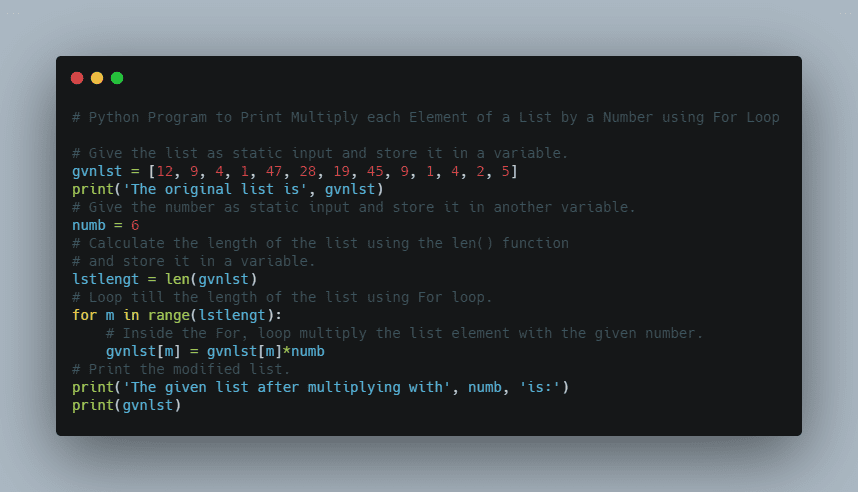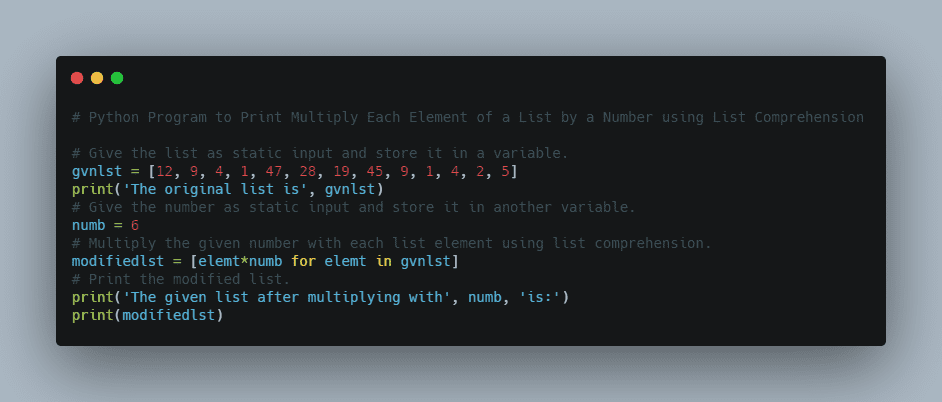# Python Program to Multiply Each Element of a List by a Number | How to Multiply Each Element in a List by a Number in Python?

Have you mastered basic programming topics of java and looking forward to mastering advanced topics in a java programming language? Go with these ultimate Advanced java programs examples with output & achieve your goal in improving java coding skills.

Multiplying each element of a list will create a new list with each value from the original list multiplied by the number specified. In this tutorial, we have explained the different methods on how to multiply each element of the list by a number in python using for loops, numpy array, list comprehension, etc. Learn the Multiplication of All Elements of a List Process by having a quick glance at the sample python programs provided below.

### Multiply Each Element of a List by a Number in Python Examples

Given a list and a number the task is to multiply each element of the given list by the given number in Python.

Example 1:

Input:

Given list = [12, 9, 4, 1, 47, 28, 19, 45, 9, 1, 4, 2, 5]
Given element =6

Output:

The original list is [12, 9, 4, 1, 47, 28, 19, 45, 9, 1, 4, 2, 5]
The given list after multiplying with 6 is:
[72, 54, 24, 6, 282, 168, 114, 270, 54, 6, 24, 12, 30]

Example 2:

Input:

Given list = [7, 1, 45, 88, 12, 65, 2, 3, 9, 7]
Given element =10

Output:

The original list is [7, 1, 45, 88, 12, 65, 2, 3, 9, 7]
The given list after multiplying with 10 is:
[70, 10, 450, 880, 120, 650, 20, 30, 90, 70]

## How to Multiply Each Element in a List by a Number in Python?

Below are the ways to multiply each element of the given list by the given number in Python.

### Method #1: Using For Loop (Static Input)

Approach:

• Give the list as static input and store it in a variable.
• Give the number as static input and store it in another variable.
• Calculate the length of the list using the len() function and store it in a variable.
• Loop till the length of the list using For loop.
• Inside the For, loop multiply the list element with the given number.
• Print the modified list.
• The Exit of the Program.

Below is the implementation:# Give the list as static input and store it in a variable.
gvnlst = [12, 9, 4, 1, 47, 28, 19, 45, 9, 1, 4, 2, 5]
print('The original list is', gvnlst)
# Give the number as static input and store it in another variable.
numb = 6
# Calculate the length of the list using the len() function
# and store it in a variable.
lstlengt = len(gvnlst)
# Loop till the length of the list using For loop.
for m in range(lstlengt):
# Inside the For, loop multiply the list element with the given number.
gvnlst[m] = gvnlst[m]*numb
# Print the modified list.
print('The given list after multiplying with', numb, 'is:')
print(gvnlst)


Output:

The original list is [12, 9, 4, 1, 47, 28, 19, 45, 9, 1, 4, 2, 5]
The given list after multiplying with 6 is:
[72, 54, 24, 6, 282, 168, 114, 270, 54, 6, 24, 12, 30]

### Method #2: Using For Loop (User Input)

Approach:

• Give the list as user input using list(),map(),input(),and split() functions.
• Store it in a variable.
• Give the number as user input using int(input()) and store it in another variable.
• Calculate the length of the list using the len() function and store it in a variable.
• Loop till the length of the list using For loop.
• Inside the For, loop multiply the list element with the given number.
• Print the modified list.
• The Exit of the Program.

Below is the implementation:

# Give the list as user input using list(),map(),input(),and split() functions.
# Store it in a variable.
gvnlst = list(map(int, input(
'Enter some random List Elements separated by spaces = ').split()))
# Give the number as user input using int(input()) and store it in another variable.
numb = int(input('Enter some random number = '))
# Calculate the length of the list using the len() function
# and store it in a variable.
lstlengt = len(gvnlst)
# Loop till the length of the list using For loop.
for m in range(lstlengt):
# Inside the For, loop multiply the list element with the given number.
gvnlst[m] = gvnlst[m]*numb
# Print the modified list.
print('The given list after multiplying with', numb, 'is:')
print(gvnlst)


Output:

Enter some random List Elements separated by spaces = 4 85 11 1 9 45 35 16 25 46 72 36 8 5 3 7
Enter some random number = 4
The given list after multiplying with 4 is:
[16, 340, 44, 4, 36, 180, 140, 64, 100, 184, 288, 144, 32, 20, 12, 28]

### Method #3: Using List Comprehension (Static Input)

Approach:

• Give the list as user input using list(),map(),input(),and split() functions.
• Store it in a variable.
• Give the number as user input using int(input()) and store it in another variable.
• Multiply the given number with each list element using list comprehension.
• Print the modified list.
• The Exit of the Program.

Below is the implementation:# Give the list as static input and store it in a variable.
gvnlst = [12, 9, 4, 1, 47, 28, 19, 45, 9, 1, 4, 2, 5]
print('The original list is', gvnlst)
# Give the number as static input and store it in another variable.
numb = 6
# Multiply the given number with each list element using list comprehension.
modifiedlst = [elemt*numb for elemt in gvnlst]
# Print the modified list.
print('The given list after multiplying with', numb, 'is:')
print(modifiedlst)


Output:

The original list is [12, 9, 4, 1, 47, 28, 19, 45, 9, 1, 4, 2, 5]
The given list after multiplying with 6 is:
[72, 54, 24, 6, 282, 168, 114, 270, 54, 6, 24, 12, 30]

### Method #4: Using List Comprehension (User Input)

Approach:

• Give the list as static input and store it in a variable.
• Give the number as static input and store it in another variable.
• Multiply the given number with each list element using list comprehension.
• Print the modified list.
• The Exit of the Program.

Below is the implementation:

# Give the list as user input using list(),map(),input(),and split() functions.
# Store it in a variable.
gvnlist = list(map(int, input(
'Enter some random List Elements separated by spaces = ').split()))
# Give the number as user input using int(input()) and store it in another variable.
numb = int(input('Enter some random number = '))
# Multiply the given number with each list element using list comprehension.
modifiedlst = [elemt*numb for elemt in gvnlist]
# Print the modified list.
print('The given list after multiplying with', numb, 'is:')
print(modifiedlst)

Output:

Enter some random List Elements separated by spaces = 4 15 19 85 36 15 25 45 39 75 82 6 4 7 9 11
Enter some random number = 10
The given list after multiplying with 10 is:
[40, 150, 190, 850, 360, 150, 250, 450, 390, 750, 820, 60, 40, 70, 90, 110]

### Multiply all Elements in a List using Numpy Array

We can use Numpy Library to multiply all the elements in a list by a number as follows

import numpy

numbers = range(10)
numpy_array = numpy.array(numbers)
new_array = numpy_array * 2

print(new_array)

Related Programs: